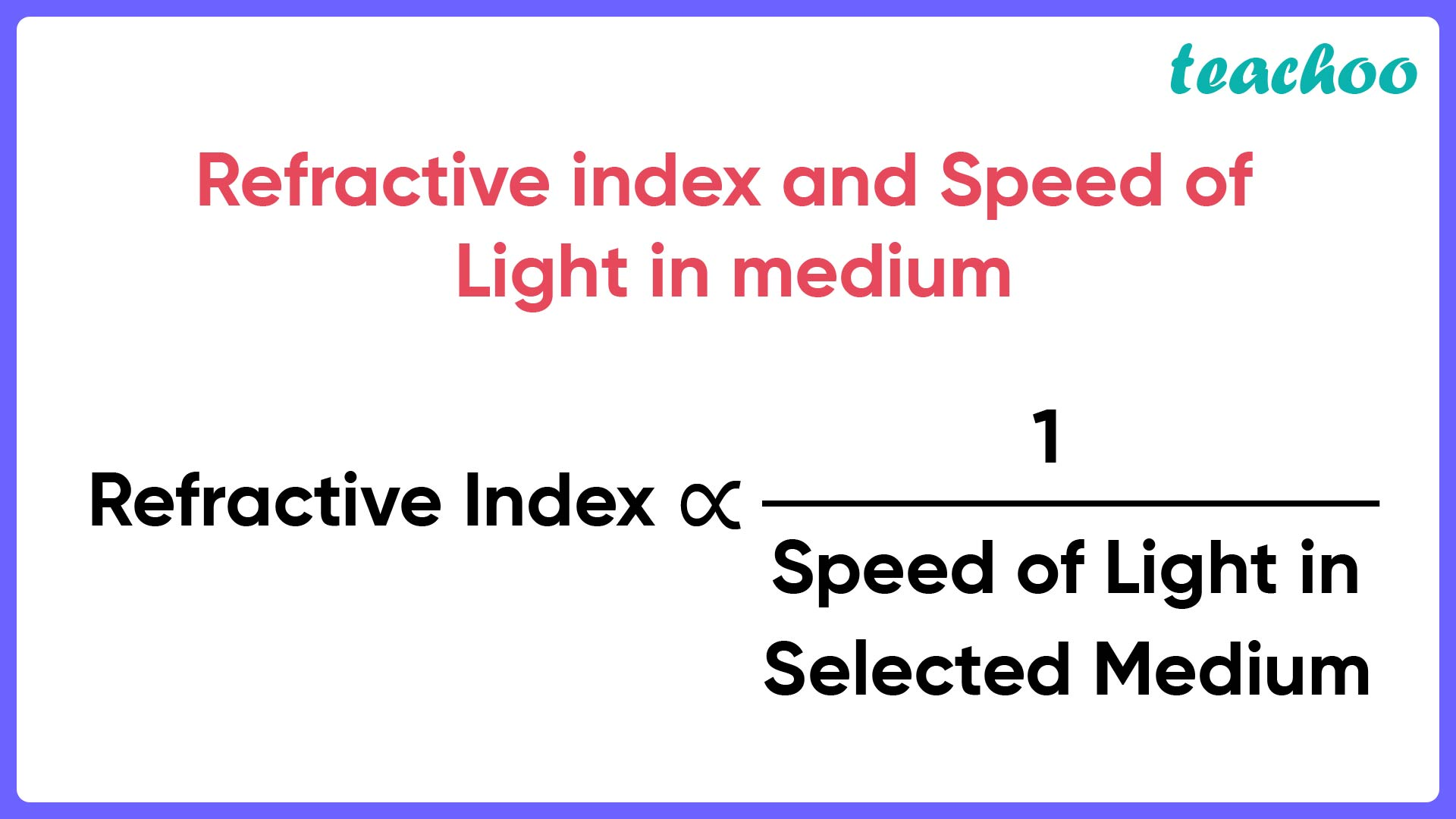Solutions - CBSE Class 10 Sample Paper for 2022 Boards - Science [MCQ]

Class 10
Solutions to CBSE Sample Paper - Science Class 10

## (D) The speed of light in glass > the speed of light in air > the speed of light in water.Now,

• Larger refractive index means denser medium
• And, the speed of light is higher in a rarer medium than a denser medium.

Thus, from our question

• Since order of Refractive Index is Glass > Water > Air
• Order of Speed of Light will be opposite, i.e. Glass < Water < Air

Thus, option C matches:The speed of light in air > the speed of light in water > the speed of light in glass.

So, the correct answer is (c)